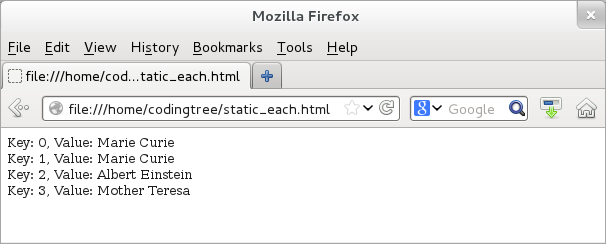## CodingBison

jQuery enables us to grab an element or elements using an identifier. Often, there can be multiple elements with the same identifier. For example, an HTML page can have multiple DOM elements belonging to a CSS class or an HTML form can have multiple text input elements. Running jQuery selection on these elements would simply return a list. Hence, handling lists is a common thing in jQuery.

When dealing with lists, one of the basic requirements is to iterate over elements of the list. To do that, JavaScript provides various looping constructs like while, do-while, for, and for-in variants. In addition to these constructs, jQuery provides its own method, .each(), to iterate over each elements. This method is available exclusively for lists returned when we select jQuery elements and cannot be used with non-jQuery JavaScript lists. And if you must use a jQuery iterator to handle non-jQuery lists, then jQuery also provides a static method, \$.each() which can iterate over plain non-jQuery objects.

### The .each() Method

The .each() method iterates on a list returned by a jQuery selector. We can pass a function to its argument and this method iterates over each element of the returned list and passes it as a parameter to the passed function. The first parameter of this function is the index of the list, starting with a value of 0. The second parameter of this function is the current element of the list.

Let us write an example that demonstrates the usage of the .each() method. The example has the following HTML structure:

``` <!doctype html>
<html>
<!-- Let us start by including the jQuery library -->
</script>

<body>
<div>Nobel Laureates:</div>

<ol>
<li>Marie Curie (Physics, 1903)</li>
<li>Marie Curie (Chemistry, 1911)</li>
<li>Albert Einstein (Physics, 1921)</li>
<li>Mother Teresa (Peace, 1979)</li>
</ol>

<script type="text/javascript" src="each.js"></script>

</body>
</html>
```

The above example includes "each.js" file (provided below) that contains the actual jQuery logic. Here, \$("li") returns a list that contains all the (four) list elements. When we call it with the .each() method, it invokes the function for each of the list element. For each iteration, the indx variable refers to the index of the current list element and the elem variable refers to the element (object) itself. Please note that jQuery (like JavaScript) uses a 0-based list index notation: the index 0 refers to the first element, the index 1 refers to the second element, and so on.

``` // Filename: each.js

// Iterate over elements returned from the \$("li") jQuery selector.
var str = "";
\$("li").each(function(indx, elem) {
str += "index-" + indx + ": "+ \$(elem).text() + "<br>";
});

// Append the text to the body element.
\$("body").append(str);
});
```

If we were to load the HTML file, then we would see the following text on the page. This output demonstrates that the .each() method walks through all list elements and at each iteration, it refers to the current element in the loop:Figure: Using jQuery's .each() Method.

As .each() iterates over each element, it changes the object context for each iteration to the current element. Thus, for each iteration, the "this" keyword refers to the current element of the list. Let us rewrite the earlier jQuery file "each.js" to use the "this" keyword to grab hold of each element in the list. With this approach, we do not need to pass any argument to the callback function. Here is the rewritten version of "each.js".

``` // Filename: each.js

// Iterate over elements returned from the \$("li") jQuery selector.
var str = "";
\$("li").each(function() {
str += \$(this).text() + "<br>";
});

// Append the text to the body element.
\$("body").append(str);
});
```

### The \$.each() Method

jQuery also provides a static method, \$.each() that can work on any generic JavaScript objects. Since JavaScript lists are also objects, \$.each() would apply to both JavaScript objects and JavaScript (arrays) lists. Please note that we should not use \$.each() method with jQuery lists -- for that, we should stick with the .each() method.

Like the .each() method of selected jQuery lists, this method also takes a function as an argument and invokes the function for each array element. Similar to the \$.each() method, jQuery provides various static methods that can work on any objects and not necessarily on jQuery selector objects.

We provide an example to illustrate the usage of the \$.each() method. The example uses a JavaScript array (or list) and passes it to the \$.each() method. Here, we pass a JavaScript array, "arr", to the \$.each() method.

``` <!doctype html>
<html>
<!-- Let us start by including the jQuery library -->
</script>

<body>
<script type="text/javascript">
// Iterate over a JavaScript array.
arr = ["Marie Curie",  "Marie Curie", "Albert Einstein", "Mother Teresa"];
str = "";
\$.each(arr, function(k, v) {
str += "Key: " + k + ", Value: " + v + "<br>";
});

\$("body").append(str);
});
</script>
</body>

</html>
```

Here is the output, when we load the page:Figure: Using jQuery's static .each() Method on a JavaScript array.